Learning Library
Who we are
Guidance

# 1.OA.A.2 Worksheets, Workbooks, Lesson Plans, and Games

#### CCSS.MATH.CONTENT.1.OA.A.2

:
"Solve word problems that call for addition of three whole numbers whose sum is less than or equal to 20, e.g., by using objects, drawings, and equations with a symbol for the unknown number to represent the problem."

These worksheets can help students practice this Common Core State Standards skill.

## Worksheets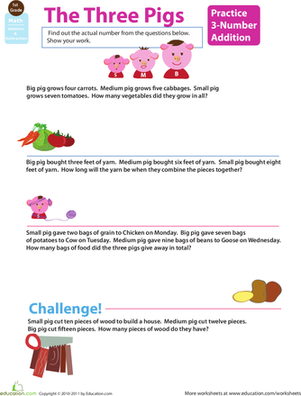Worksheet
If your child is confident in his addition skills, challenge him to tackle addition with three numbers.
Math
Worksheet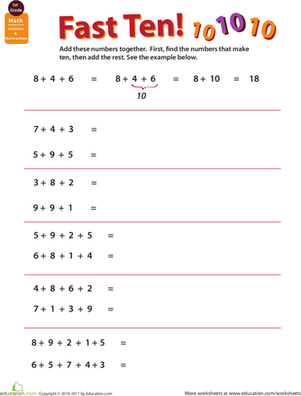Worksheet
Challenge your child to add three, four, and five numbers together. He may find the task daunting, but there's a trick to add multiple numbers that will help!
Math
Worksheet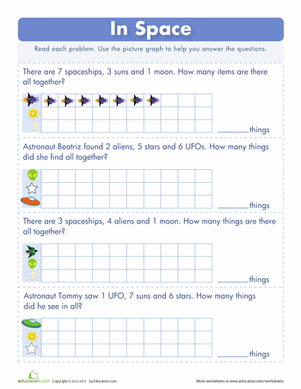Worksheet
Explore some stellar math concepts with this worksheet on adding multiple digits. Fill in the pictograph to help you visualize the problem first.
Math
Worksheet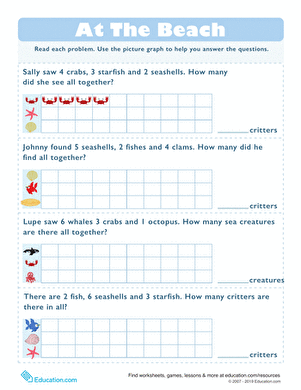Learning Charts
Worksheet
Learning Charts
Take math practice to the beach with this intro to pictographs! Practice drawing each word problem on the chart.
Math
Worksheet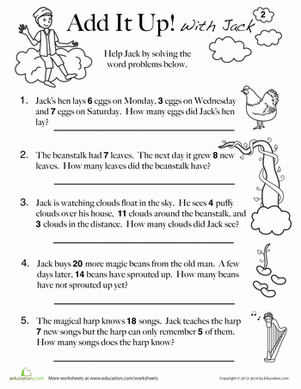Jack and the Beanstalk Math
Worksheet
Jack and the Beanstalk Math
Jack and the Beanstalk math means counting beans, and adding up how many songs the magic harp knows how to play in word problems for young kids.
Math
Worksheet

## Lesson PlansActive Math
Lesson plan
Active Math
Have a class that can't sit still long enough to practice their addition? This activity puts math and PE together to help your kids add up to 20 while being active!
Math
Lesson plan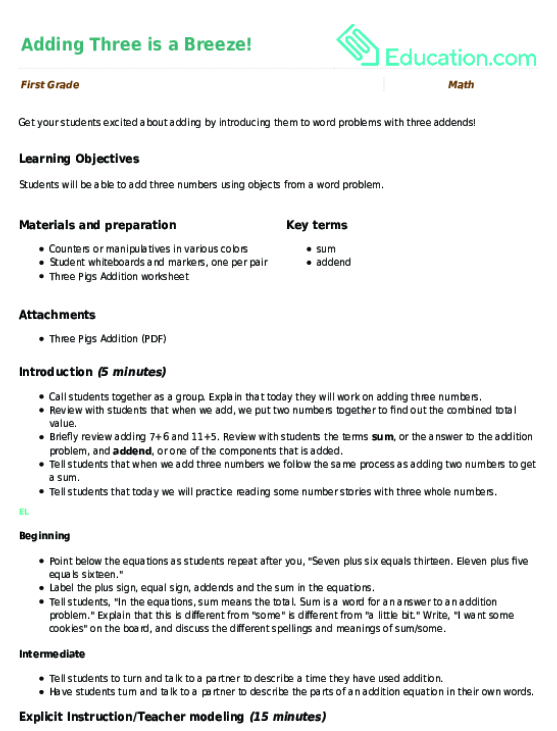Adding Three is a Breeze!
Lesson plan
Adding Three is a Breeze!
Get your students excited about adding by introducing them to word problems with three addends!
Math
Lesson planHow'd You Get That Many?
Lesson plan
How'd You Get That Many?
Drawing on various problem solving techniques, students will challenge themselves to solve word problems involving three whole numbers. Problem solving, critical thinking, and real life applications? This lesson has it all.
Math
Lesson planEaster Word Problems
Lesson plan
Easter Word Problems
It’s time to start adding and subtracting with word problems! In this Easter-themed lesson, students will get to practice their reading and math skills as they figure out how to write equations and solve addition and subtraction word problems.
Math
Lesson plan

## Workbooks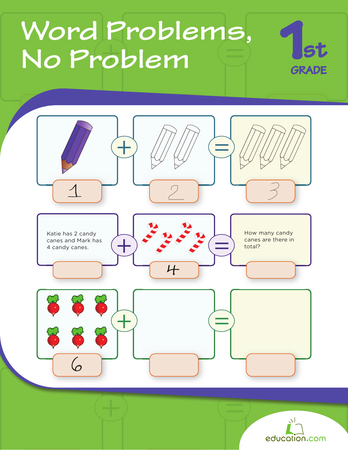Word Problems, No Problem
Workbook
Word Problems, No Problem
Get a great introduction to word problems with this round up of practice pages. Kids will review addition and subtraction from a different approach, and learn how math can apply to the real world.
Math
Workbook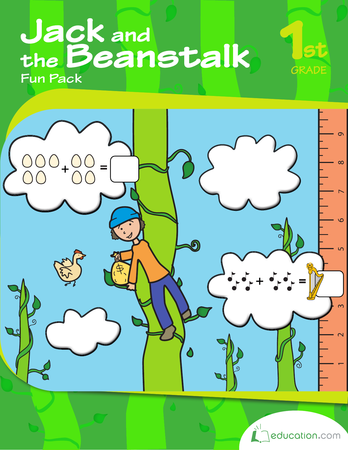Jack and the Beanstalk Fun Pack
Workbook
Jack and the Beanstalk Fun Pack
This workbook tells the story of Jack and the Beanstalk. Read, count and measure along with Jack!
Workbook

## Games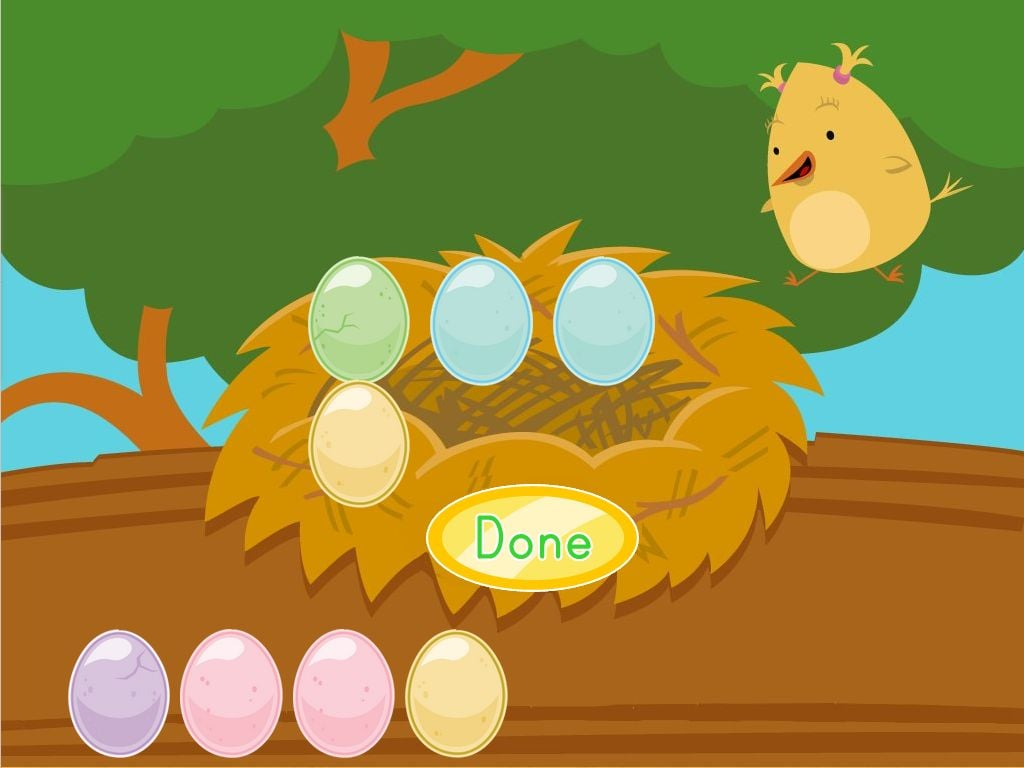Adding Eggs with Birdee
Game
Adding Eggs with Birdee
Your kid will add the right number of eggs to the nest to practice addition facts.
Kindergarten
Math
Game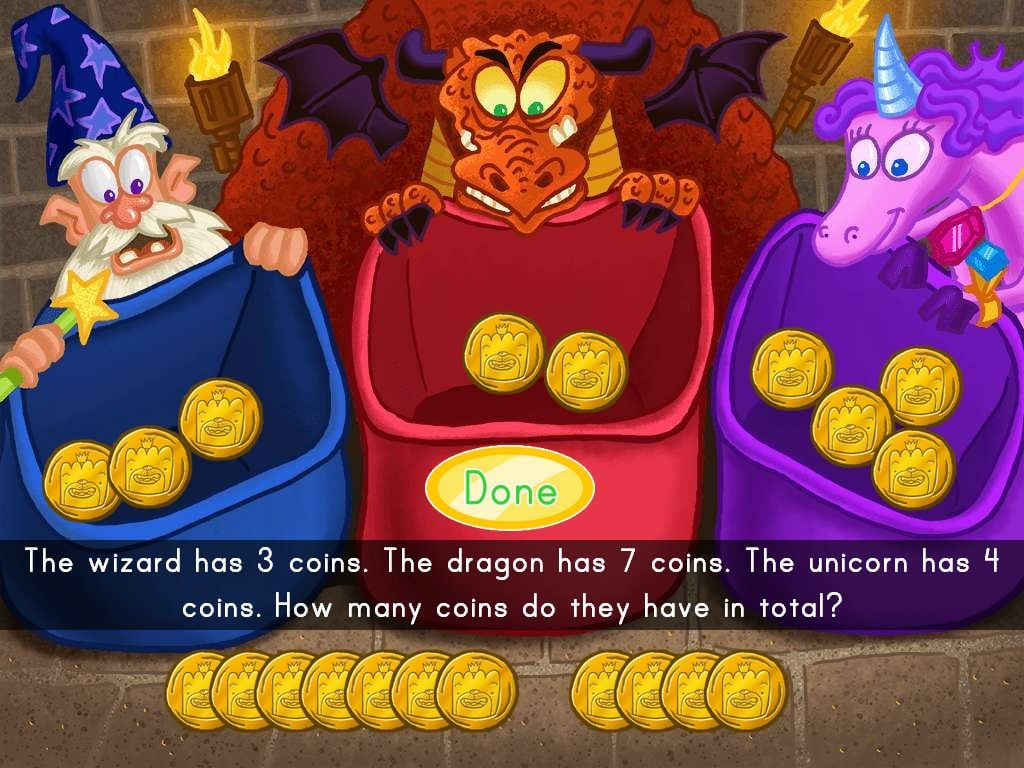Add Up the Fantasy Coins
Game
Add Up the Fantasy Coins
Kids solve a multi-step word problem by counting out the right number of coins.